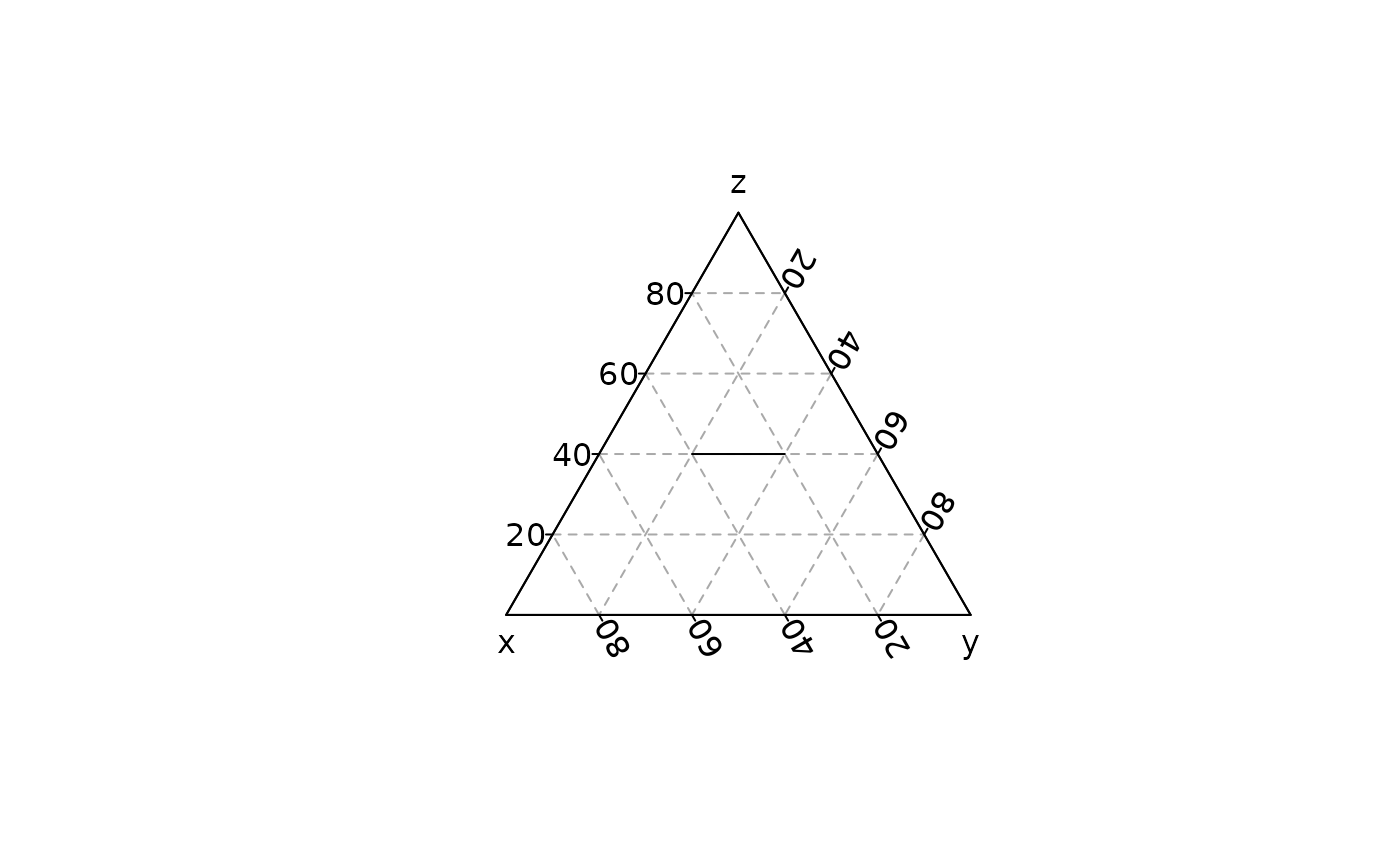Draw line segments between pairs of points.

## Usage

ternary_segments(x0, y0, z0, ...)

# S4 method for numeric,numeric,numeric
ternary_segments(x0, y0, z0, x1 = x0, y1 = y0, z1 = z0, ...)

## Arguments

x0, y0, z0

A numeric vector giving the x, y and z ternary coordinates of points from which to draw.

...

Further graphical parameters (see graphics::par()) may also be supplied as arguments, particularly, line type, lty, line width, lwd and color, col. Also the line characteristics lend, ljoin and lmitre.

x1, y1, z1

A numeric vector giving the x, y and z ternary coordinates of points to which to draw.

## Value

ternary_segments() is called it for its side-effects.

graphics::segments()

Other geometries: ternary_arrows(), ternary_crosshairs(), ternary_lines(), ternary_points(), ternary_polygon(), ternary_text()

N. Frerebeau

## Examples

## Add segments
ternary_plot(NULL, panel.first = ternary_grid())
ternary_segments(x0 = 40, y0 = 20, z0 = 40,
x1 = 20, y1 = 40, z1 = 40)